## Example Questions

### Example Question #1 : How To Find The Endpoints Of A Line Segment

The midpoint of line segment AB is (2, -5).  If the coordinates of point A are (4, 4), what are the coordinates of B?

(3, -0.5)

(6, 13)

(0, -13)

(6, 14)

(0, -14)

(0, -14)

Explanation:

The fastest way to find the missing endpoint is to determine the distance from the known endpoint to the midpoint and then performing the same transformation on the midpoint.  In this case, the x-coordinate moves from 4 to 2, or down by 2, so the new x-coordinate must be 2-2 = 0.  The y-coordinate moves from 4 to -5, or down by 9, so the new y-coordinate must be -5-9 = -14.

An alternate solution would be to substitute (4,4) for (x1,y1) and (2,-5) for (x,y) into the midpoint formula:

x=(x1+x2)/2

y=(y1+y2)/2

Solving each equation for (x2,y2) yields the solution (0,-14).

### Example Question #1 : Midpoint Formula

Point A is (5, 7).  Point B is (x, y).  The midpoint of AB is (17, –4). What is the value of B?

(12, –11)

(22, –9)

(8.5, –2)

(29, –15)

(29, –15)

Explanation:

Point A is (5, 7). Point B is (x, y). The midpoint of AB is (17, –4). What is the value of B?

We need to use our generalized midpoint formula:

MP = ( (5 + x)/2, (7 + y)/2 )

Solve each separately:

(5 + x)/2 = 17 → 5 + x = 34 → x = 29

(7 + y)/2 = –4 → 7 + y = –8 → y = –15

Therefore, B is (29, –15).

### Example Question #1 : How To Find The Endpoints Of A Line Segment

Line segment AB has an endpoint, A, located at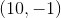, and a midpoint at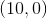. What are the coordinates for point B of segment AB?

The second endpoint cannot exist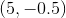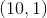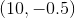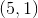Explanation:

With an endpoint A located at (10,-1), and a midpoint at (10,0), we want to add the length from A to the midpoint onto the other side of the segment to find point B. The total length of the segment must be twice the distance from A to the midpoint.

A is located exactly one unit below the midpoint along the y-axis, for a total displacement of (0,1). To find point B, we add (10+0, 0+1), and get the coordinates for B: (10,1).

### Example Question #4 : How To Find The Endpoints Of A Line Segment

Solve each problem and decide which is the best of the choices given.

What is the distance between the points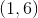and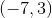on a standard coordinate plane?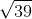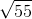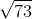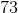Explanation:

Make a triangle. The points are 8 units apart on the-axis, andunits apart on the-axis. Then use the Pythagorean Theorem to find the distance of the hypotenuse, which ends up being.

Another way to solve this problem is to use the distance formula,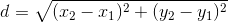Plugging in the two points we get,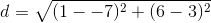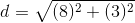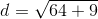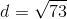### All SAT Math Resources